By going through these CBSE Class 11 Maths Notes Chapter 11 Conic Sections Class 11 Notes, students can recall all the concepts quickly.

## Conic Sections Notes Class 11 Maths Chapter 11

Conic : Let T be a fixed line and F be a fixed point (not on line T).
Let P be any point in the plane of the line T and the point F. Then, the set of all points P, such that | FP | = e | PM |, where M is the foot’ of perpendicular from P on the line l and e > 0 is . a fixed real number is called a conic.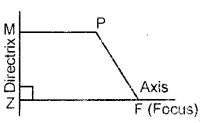Notes :

1. The fixed point F is called focus of the conic and the fixed line T is called directrix of the conic associated with F.
2. The positive real number e is called eccentricity of the conic.
3. A line through the focus F and perpendicular to the directrix l is called axis of the conic.
4. The point of intersection of the conic and its axis is called
vertex.
5. A conic with eccentricity ‘e’ is called
(i) a parabola, if e = 1. (ii) an ellipse, if e < 1. (iii) a hyperbola if e > 1..

Parabola : Let T be a fixed line and F be a fixed point (not on 1). Let P be any point in the plane of line l and the point F. Let M be the foot of perpendicular from P on the line l. Then, the set of all points P such that | FP | = | PM | is called a parabola.

Equation of the Parabola (Standard Form): The equation of the parabola in the standard form is y2 = 4ax.

Latus Rectum : The latus rectum of conic is the chord passing through the focus and perpendicular to its axis.

Length of Latus Rectum : The length of the latus rectum of the parabola y2 = 4ax is 4a.

Focal Distance : The distance of any point on parabola from the focus is called focal distance of that point.

Focal Chord : Any chord that passes through the focus of the parabola is called a focal chord of the parabola.

The focal distance of a point P(x1, y1 of the parabola y2 = 4ax is a + x1

Main Facts about Four Standard Forms of Parabola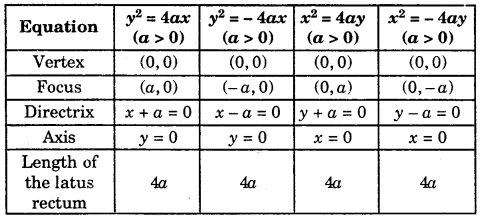Ellipse : Let l be a fixed line, F be a fixed point (not on l). Let P be any point in the plane of the line l and the point F.Let M be the foot of perpendicular from P on the line l.
Then, the set of all points P such that | FP | = e | PM | (0 < e < 1) is called an ellipse.

Notes : 1. The fixed line is called directrix of the ellipse.
2. The fixed point F is called focus of the ellipse.
3. The positive real number e < 1 is called eccentricity of the ellipse.

Standard form of an Ellipse : The equation of the ellipse in the standard form is $$\frac{x^{2}}{a^{2}}+\frac{y^{2}}{b^{2}}$$ = 1, where b2 = a2( 1 – e2) = a2 – a2e2.
Putting ae = c. Therefore, c2 = a2 – b2.

Note: The ellipse $$\frac{x^{2}}{a^{2}}+\frac{y^{2}}{b^{2}}$$ = 1 has two foci, namely (ae,o) and (- ae, 0) and their respective directrices are x – $$\frac{a}{e}$$ = 0 and x + $$\frac{a}{e}$$ = 0.

Main Facts about two Standard forms of Ellipse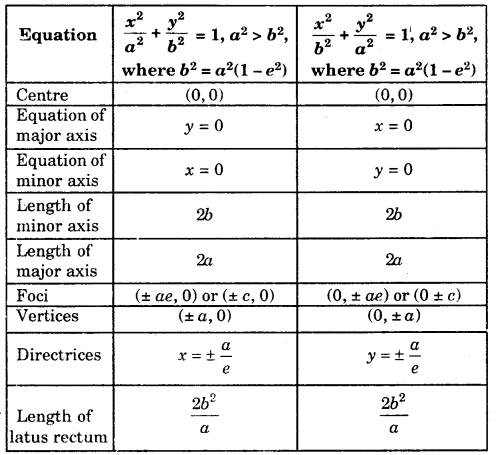Notes : 1. The focal distances of any point (x1, y1) of the ellipse $$\frac{x^{2}}{a^{2}}+\frac{y^{2}}{b^{2}}$$ = 1 are a ± ex1
2. Sum of the focal distances of a point of the ellipse is equal to length of the major axis.

Hyperbola: Let l be a fixed line and F be a fixed point (not on l). Let P be any point in the plane of the line l and the point F. Let M be the foot of perpendicular from P on the line l. Then, the set of all points P such that | FP | = e | PM | (e > 1) is called a hyperbola.

2. Sum of the focal distances of a length of the major axis.

Hyperbola: Let l be a fixed line and F be a fixed point (not on l). Let P be any point in the plane of the line l and the point F. Let M be the foot of perpendicular from P on the line l. Then, the set of all points P such that | FP | = e | PM | (e > 1) is called a hyperbola.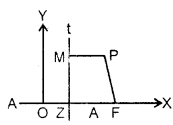Standard form of Hyperbola : The equation of a hyperbola in the standard form is $$\frac{x^{2}}{a^{2}}-\frac{y^{2}}{b^{2}}$$ = 1, where b2 = a2(e2 – 1). Putting ae -c, b2 – c2 – a2 or c2 – a2 + b2.

Notes: 1. The hyperbola $$\frac{x^{2}}{a^{2}}-\frac{y^{2}}{b^{2}}$$ = 1 has two foci namely (- ae, 0) and (ae, 0) with two respective directrices as x + $$\frac{a}{e}$$ = 0 and x – $$-\frac{a}{e}$$ = 0

2. | ex1 – a | and | ex1 + a | are the focal distances of any point (x1, y1) on the hyperbola $$\frac{x^{2}}{a^{2}}-\frac{y^{2}}{b^{2}}$$ = 1.

Main facts about two standard forms of hyperbola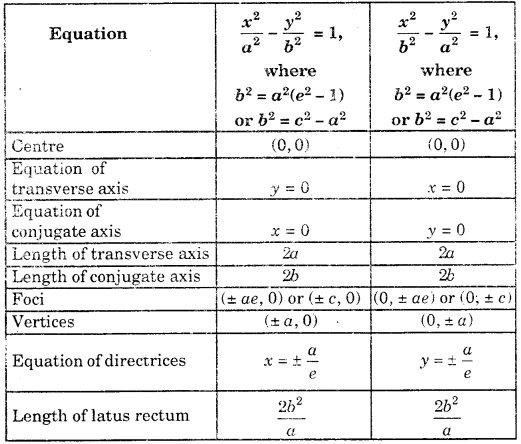Circle : The locus of a point which moves in a plane such that its distance from a fixed point, in the plane, is always a constant, is called a circle. The fixed point is called the centre and the constant distance is called the Radius of the circle.

Equation of the Circle : The equation of a circle with centre (h, k) and radius V’ is given by (x – h)2 + (y – k)2 = r2.
If the centre of a circle is origin and radius is ‘r’ then its equation is x2 + y2 = r2.

General Equation of a Circle : The general equation of a circle is x2 + y2 + 2gx + 2fy + c = 0.

Its centre is (-g,-f) and radius = $$\sqrt{g^{2}+f^{2}-c}$$

Note : 1. If g2 + f2 – c = 0, then radius = 0.
In this case, x2 +y2 + 2gx + 2fy + c = 0 represents a circle whose radius is zero. Such a circle is called a Point Circle, [i.e., it represents the point (-g, -f)].

2. If g2 + f2 – c < 0, then radius is non-real. In this case, x2 +y2 + 2gx + 2fy + c = 0 represents the empty set. 3. If g2 + f2 – c > 0, then radius is real and therefore,
x2 + y2 + 2gx + 2fy + c = 0 represents a circle whose centre is at the point (- g,- f) and radius = $$\sqrt{g^{2}+f^{2}-c}$$. (where g2 + f2 – c > 0).

Conditions for an Equation to Represent a Circle :

(i) It should be a second degree equation in x and y.
(ii) Coefficient of x2 = Coefficient of y2 ≠ 0.
(iii) It should not contain any term of the product xy.

Method to find the centre, radius of a circle :

(i) Write the equation of the circle with the coefficient of x2 and y2 being unity.
(ii) Compare this with x2 + y2 + 2gx + 2fy + c = 0 and find the value of g,f and c.
(iii) Then, (-g,-f) is the centre and $$\sqrt{g^{2}+f^{2}-c}$$ is the radius of the circle.